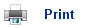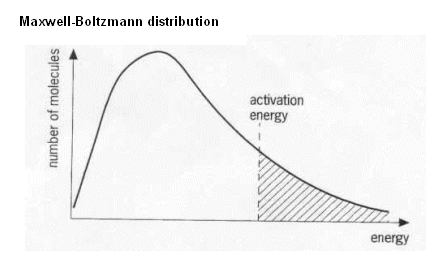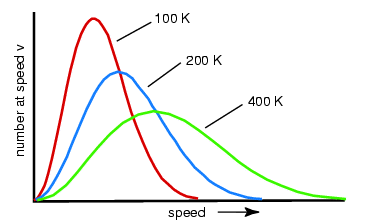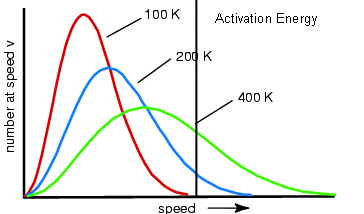# Rates of Reaction

## Collision Theory

• The collision theory can be used to explain the factors affecting rates of reaction.
• The basic theory behind the collision theory is that chemicals react when their particles successfully collide.
• Take for instance the reaction between hydrogen and nitrogen to produce ammonia.
• The collision theory states that the reaction occurs when the hydrogen particles and nitrogen particles successfully collide.
• The more frequently they collide, the faster the rate of reaction.

## Effect of Pressure

• The higher the pressure, the more particles there are per volume. If there are more particles in a space, then there will be more collisions within a given time period. This means that the rate of reaction will be higher.

## Effect of Temperature

• When the temperature increases, the particles gain kinetic energy and move around faster. This results in more collisions.
• The speed of the molecules is proportional to the square root of the temperature (K).
• However, it’s not only how frequently the particles collide that matters, but also how much energy they collide with.
• The minimum energy needed for the reaction to occur is called the activation energy.
• Unless the particles collide with the energy equal to the activation energy, they just bounce off each other, and remain un-reacted.
• This is what happens most of the time during reactions, for example when N2 and H2 react at 300 K, only 1 in 1011 collisions results in a successful reaction.
• The collision theory states that reactions occur when molecules collide with a certain amount of energy. The more frequent these reactions, the faster the reaction.

## Distribution of Energies

• At a temperature, the kinetic energy of the molecules varies; the different molecules move at different speeds.
• Some molecules have high kinetic energies, some have medium, and some have low kinetic energies.
• The Maxwell-Boltzmann distribution can be used to represent the distribution of kinetic energies.• As the temperature increases, the molecules kinetic energy increases; they move around faster.
• There is still a distribution of energies at higher temperatures, however a greater proportion of molecules have higher energies.• These may look irrelevant to reaction rates; however they do have a purpose.
• If we add the activation energy to the distribution, then we can see how much of the distribution will result in successful collisions (below).• The molecules to the right of the activation energy line can react, whereas the molecules to the left of the line are unable to.
• Notice that the distributions with higher temperatures have more of the graph to the right of the line; therefore more of the molecules react.
• This can be summarized by saying that reactions go faster at higher temperatures because a larger proportion of molecules have the required activation energy needed to react.
• Increasing the temperature may not make a difference to the energy of each individual molecule, but it makes a big difference to the proportion of the molecules.

## Useful books for revision:

Revise AS Chemistry for Salters (Written by experienced examiners and teachers of Salter's chemistry)Revise AS Chemistry for Salters (OCR) (Salters Advanced Chemistry)Home